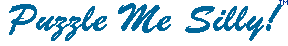Math Puzzles

Math Teasers

Exclusive to Puzzle Me Silly, Math Teasers are a cross between a math square and a cryptogram. Lots of fun! Brought to you by Zhivko Stoyanov from Bulgaria.

Click here for the collection!

Math Squares

Fill in the missing numbers to get the number at the end of the row!

 Math Square 1 Math Square 2 Math Square 3 Math Square 4 Math Square 5 Math Square 6 Math Square 7 Math Square 8 Math Square 9 Math Square 10
Copyright © 2012 Variety Games Inc.